# Θ Theta Criteria

## Multivariate Analysis

By Steve Halitsky and Edward Halitsky# I. Existing Statistical Methods for Multivariate Data Processing

## Multivariate General Linear Hypothesis

There are three major mathematical and statistical software packages to process multivariate data:

§   MATLAB® 

§   SAS® 

§   SPSS® 

These software packages are based on Multivariate General Linear Hypothesis(MGLH) :

§   All dataset variables are linear

§   Relationships models are linear series of weighted terms.

The MGLH is implemented using the following procedures:

§  Multiple Regression

§  Discriminant Function Analysis

§  Canonical Analysis

§  Principle Components Analysis

§  Formal linear algebra methods

We will now discuss these procedures in detail.

## Multiple Regression Equation

y = b1x1 + b2x2 + ... + bnxn + c

In this equation, y is a dependent variable, bi - regression coefficients and xi - independent variables. This equation evaluates y variance proportion at a significant level and xi relative predictive importance. This method evaluates dependent variable based on independent variable values.

## Discriminant Function Analysis

This method determines which variables discriminate between two or more groups on covariance matrix of group variances and

co-variances. Then one of the test statistics for eigenvalue analysis, such as Wilks' Lambda, is used. This method is identical to multivariate analysis of variance or MANOVA. For several groups, additional Discriminant functions can be used.

## Canonical Analysis

This method uses optimal variables combination for multiple group Discriminant analysis. The first function is the most informative description, the second is second most, and so on. The functions ought to be independent or orthogonal. The canonical correlation analysis is based primarily on canonical roots or eigenvalues.

## Factor Structure Method

This method analyzes correlations of variables and interpretes the Discriminant functions' values. This method places heavy emphasis on results interpretation and will not be reviewed here.

## Principle Components Analysis (PCA)

This method has been used to estimate the dataset variance in terms of principle components. The method goals are to reduce data dimensionality, define the most informative components and noise filtering. The standard normalization procedure removes noise, stabilizes the data. Regrettably, this method has limited efficiency as data structure identification tool. The PCA defines mutually-orthogonal or uncorrelated projections set. For square and symmetric matrix with ordered eigenvalues, the first principal component direction coincides with 1st eigenvector direction. The second principal component direction coincides with direction of 2nd eigenvector direction. The procedure iterates until satisfactory accuracy has been achieved.

For symmetric matrix, the eigenvalue and eigenvectors can be found by a Householder reduction procedure and QL algorithm.  For

non-square or non-symmetric data matrix A, the singular value decomposition U V' of A can be formed. Here matrix V contains the eigenvectors, and the squared diagonal matrix U contains the eigenvalues , .

## Formal Linear Algebra Methods

These methods use various norms, determinant, trace and condition to evaluate the matrices distance. Nearly all of those criteria can be represented as various functions of eigenvalues , .

## Theta Criteria

According to Spectral and Hilbert Theorems, the whole sets of eigenvalues & eigenvectors or eigenvalues & eigenfunctions fully describe matrix or operator. Our methods (Theta Criteria) are constructed from whole sets of eigenvalues & eigenvectors or eigenvalues & eigenfunctions. In this scenario, Theta Criteria methods are more optimal for multivariate applications than existing methods. We studied the Theta Criteria in detail and found these methods to be more precise and accurate than existing methods , .

Let us assume that Spectral Theorem conditions are fulfilled and symmetrical operator / matrixcan be diagonalized. Also, orthonormalized basis ofexists consisting of its eigenvectors.

In addition, each eigenvalue ofis real.

Letand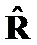be symmetrical matrices or operators. Let us construct set of criteria, which can converge on. Such criteria will reflect the geometrical changes on some the elements of,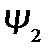or.

Let evaluate 1st differences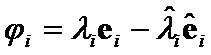between weighted eigenvectors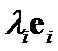and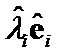. Their Euclidean norm, or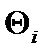criteria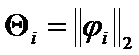can serve as closeness criteria between eigenpairsand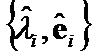(Figure 1.1.)  Analogously,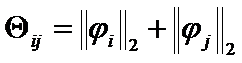can be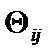criteria between pairs of eigenpairs,and,. At last,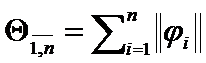be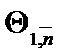criteria on all eigenpairs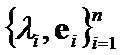.

## Figure 1.1: Theta Criteria Properties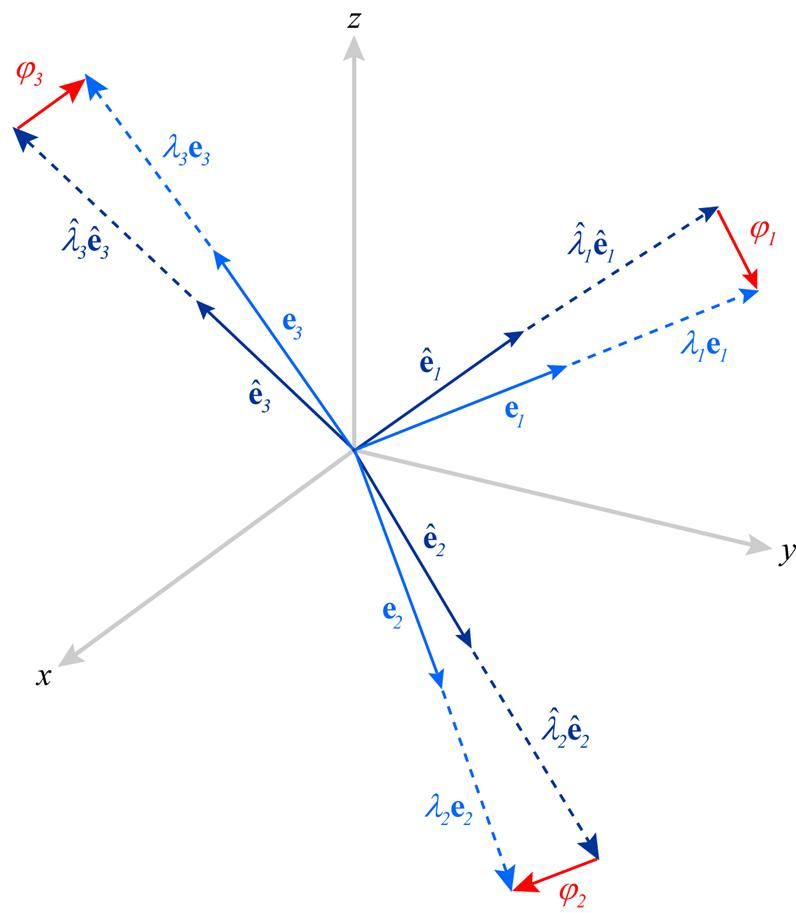We have found that Theta Criteria are norms. These methods are positive, homogeneous, positively defined and satisfy triangle inequality. The Theta Criteria can be transformed to matrix norm and trace differences.

We formulated distinction types hypotheses for positively defined matricesand. Then we evaluated accuracy of Theta Criteria andorfor very close matrices and for ill-defined matrices. Several Theta Criteria were significantly more accurate thanor. Further research is required to obtain functional representation between distinction hypotheses types and Theta Criteria optimal type(s).

## Summary

Existing application for multivariate data set processing, such as MATLAB® , SAS®  and SPSS®  utilize Multiple Regression Procedure, Discriminant Function Analysis, Canonical Analysis and Principle Components Analysis. Those methods are appropriate for initial stage of data analysis when distinction hypotheses about specific application are not formulated or not adequately described.

If distinction hypotheses were established, then formal linear algebra methods or Theta Criteria can be applied for in-depth application analysis.

The formal linear algebra methods are straightforward by utilizing only matrices' eigenvalues. If the application accuracy specifications are moderate, then these methods will be sufficient.Regrettably, formal linear algebra methods have limited accuracy for complex or ill-defined applications.

If, however, the multivariate application is ill-defined or requires high accuracy, then Theta Criteria deserve serious consideration.

## References:

1. MATLAB® USER GUIDE: www.mathworks.com/access/helpdesk/help/techdoc/data_analysis/data_analysis.html
2.   SAS® / STAT® User Guide www.id.unizh.ch/software/unix/statmath/sas/sasdoc/stat/
3. SPSS® / SCS® Documentation Guide
www.spss.com/spss/data_analysis.htm
4. L. Lebart, A. Morineau and K. Warwick (1984), Multivariate Descriptive Statistical Analysis, New York: John Wiley & Sons, Inc.
5. G. Golub, C. Van Loan (1996) Matrix Computations: The John Hopkins University Press.
6. G. Stewart (1998), Matrix Decompositions, Philadelphia: SIAM.
7. S. Lang (1993), Real and Functional Analysis, New York, Berlin: Springer-Verlag
8. K. Mardia, J. Kent and J. Bibby (1979), Multivariate Analysis, London: Academic Press.
9. V. Vasil'ev, V. Halitsky (same person as S. Halitsky) and V. Revenko. Estimation of Correlations between Groups of Parameters of a Multidimensional Plant. Soviet Automat. Control. 1975 No. 2, 9-15.